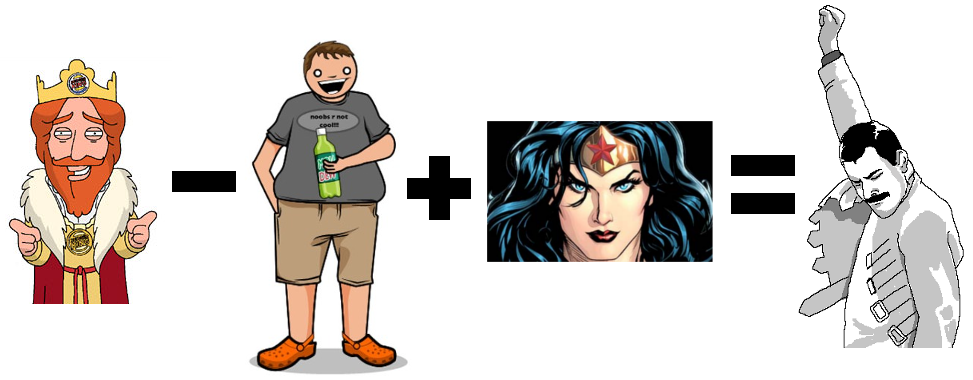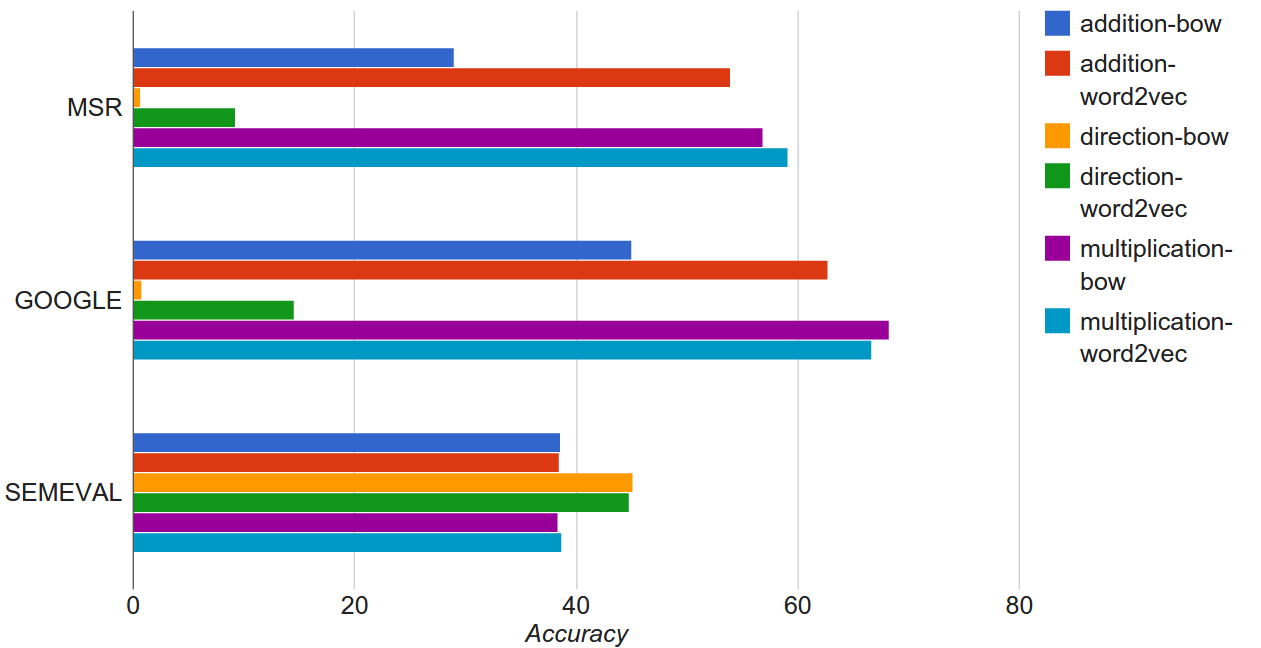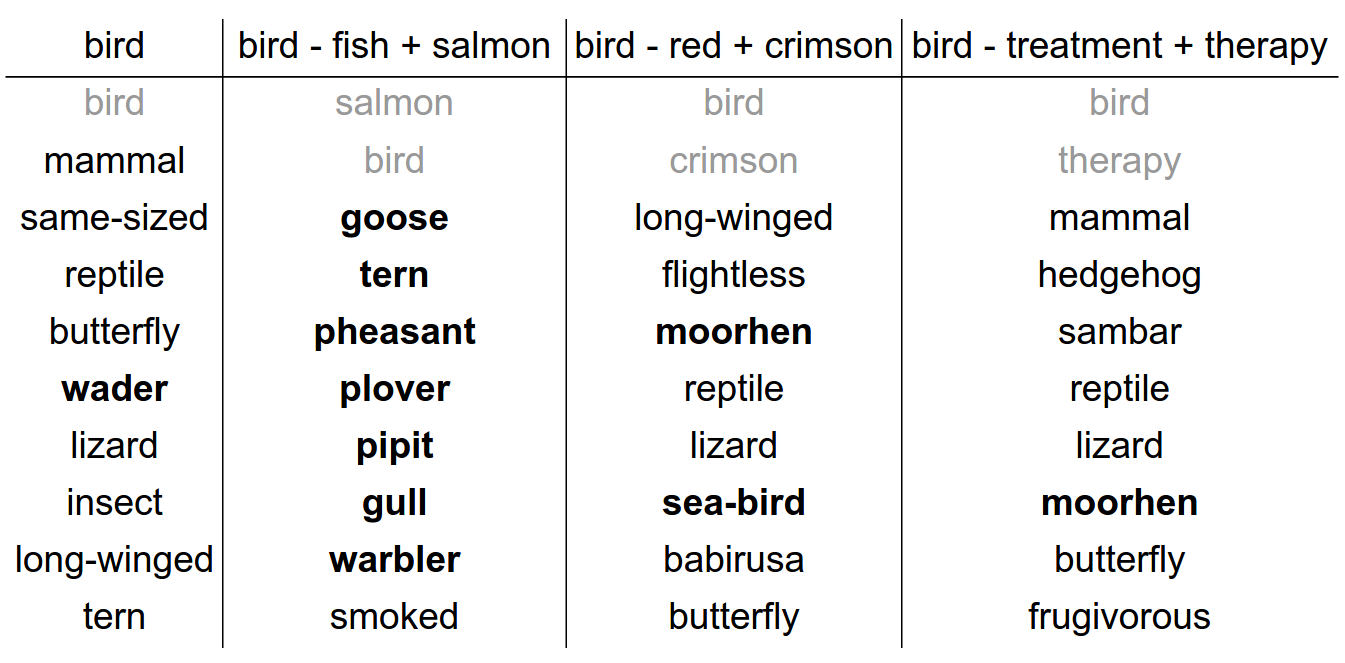# Linguistic Regularities in Word Representations

In 2013, Mikolov et al. (2013) published a paper showing that complicated semantic analogy problems could be solved simply by adding and subtracting vectors learned with a neural network. Since then, there has been some more investigation into what is actually behind this method, and also some suggested improvements. This post is a summary/discussion of the paper “Linguistic Regularities in Sparse and Explicit Word Representations“, by Omer Levy and Yoav Goldberg, published at ACL 2014.

The task under consideration is analogy recovery. These are questions in the form:

a is to b as c is to d

In a usual setting, the system is given words a, b, c, and it needs to find d. For example:

‘apple’ is to ‘apples’ as ‘car’ is to ?

where the correct answer is ‘cars’. Or the well-known example:

‘man’ is to ‘woman’ as ‘king’ is to ?

where the desired answer is ‘queen’.

While methods such as relation extraction would also be completely reasonable approaches to this problem, the research is mainly focused on solving it by using vector similarity methods. This means we create vector representations for each of the words, and then use their positions in the high-dimensional feature space to determine what the missing word should be.

## Vector Spaces

As the first step, we need to create feature vectors for each word in our vocabulary. The two main ways of doing this, which are also considered by this paper, are:

1. BOW: The bag-of words approach. We count all the words that a certain word appears with, treat the counts as features in a vector, and weight them using something like positive pointwise mutual information (PPMI).
2. word2vec: Vectors created using the word2vec toolkit. Low-dimensional dense vector representations are learned for each word using a neural network.

If you want to learn more details about these models, take a look at an earlier post about comparing both of these models.

## Analogy Artihmetic

We have the vectors, but how can we find the correct words in the analogy task?

The idea of Mikolov et al. (2013) was that the vector offset of vectors should reflect their relation:

$$a – b \approx c – d$$
$$man -woman \approx king -queen$$

We can rearrange this to find d:

$$d \approx c – a +b$$
$$queen \approx king -man +woman$$

Therefore, we can calculate the vector for $$c – a + b$$, compare it to the vectors of all words in our vocabulary, and return the highest-scoring word as the answer.

$$d_w = arg max_{d_w’ \in V}(cos(d’, c – a +b))$$

I’m using $$d_w$$ here to denote the actual word, whereas the $$d$$ stands for the vector representation of $$d_w$$. $$V$$ is our whole vocabulary. $$cos()$$ is the cosine similarity between two vectors. Let’s refer to this as the addition method.An alternative to this is to consider the directions of the offset directly. We find the word that has the most similar offset (in terms of direction), compared to the offset of the sample words.

$$d_w = arg max_{d’_w \in V}(cos(a – b, c – d’))$$

It only looks at the direction of the relational step, ignoring the actual magnitude of this step. We’ll see later that sometimes this is good, sometimes not so much. We’ll refer to this method as direction. Apparently this method was actually used by Mikolov in some experiments, although it wasn’t included in the paper.

Now onto the modification proposed by Levy & Goldberg (2014). The addition method basically looks for a word that is similar to c and b, and not similar to a, with each contributing equally. This can lead to cases where one large term dominates the equation, especially if the magnitudes of the similarities are very different. Therefore, the authors have proposed that instead of adding these similarities, they could be multiplied:

$$d_w = arg max_{d’_w \in V}\frac{cos(d’, c) cos(d’, b)}{cos(d’, a) + \epsilon}$$

If the vectors are normalised (unit length), then this formula actually becomes a multiplication of their scalar products:

$$d_w = arg max_{d’_w \in V}\frac{(d’ \cdot c) \times (d’ \cdot b)}{(d’ \cdot a) + \epsilon}$$

$$\epsilon$$ is set to 0.001 to avoid division by zero. Also, the formula doesn’t handle negative similarity scores, whereas cosine (and the scalar product) can be negative. Therefore, the values are transformed into a positive range by doing $$x_{new} = (x + 1)/2$$. We’ll refer to this as the multiplication method.

## Evaluation

The alternative methods are evaluated on the task of analogy recovery, using three different datasets:

1. The MSR dataset containing 8,000 analogy questions.
2. The GOOGLE dataset with 19,544 analogy questions.
3. The SEMEVAL dataset, covering 79 distinct relation types.

In the MSR and GOOGLE datasets, the system needs to find the correct answer from an open vocabulary. In contrast, the SEMEVAL task provides candidates for each relation that need to be reranked. Accuracy is used as the evaluation metric on all datasets.

The word vectors were trained on 1.5 billion words of English Wikipedia. The vocabulary was restricted to contain only words that occurred at least 100 times in the corpus, resulting in 189,533 words.

## ResultsSome observations based on the results:

• The multiplication method outperforms the additon method on two tasks, and gives comparable results on the third one.
• The direction method performs horribly on the MSR and GOOGLE tasks, but gives the best results on SEMEVAL. This is because the method only looks at the direction of the relation, but doesn’t care if the result is actually positioned very far in the semantic space. The SEMEVAL task restricts the candidates to a small list, and the method is able to pick the best option, but doesn’t perform as well on open vocabulary.
• The BOW model is generally worse than word2vec when using the addition method, but is comparable to the best results when using multiplication. This indicates that the property of linguistic regularities applies to both classical BOW and neural network representations.

## Discussion

Based on my experience with applying different vector operations to discover linguistic regularities, I’d like to point out two observations.

First, while the ability to recover semantic relations in such a way is remarkable, it is not quite as impressive as it seems at first glance. Let’s take the well-known example of king – man + woman = queen. Depending on the parameters I used, several vector spaces I built actually gave queen as the most similar word to king, with no vector operations needed. This makes sense, because king and queen are semantically very related, and probably occur in very similar contexts. But it also means that (woman – man) only needs to be a zero vector or some small random noise in order to give the correct answer. I’m not saying this is a major factor in these experiments, but I would encourage future work to also report baselines without using any vector operations.

Second, I observed that the quality of these regularities highly depends on the examples that are provided, and how they relate to the query word. For my work (Rei & Briscoe, 2014) I looked at hyponym relations, and below are some examples when trying to find hyponyms for bird.The greyed out terms are the query terms, and it is common practice to ignore these in the results; the bold results are correct answers. As you can see, just the plain vector similarity returns one bird species correctly in the top results. When using (salmon,fish) as the example pair, the system is quite good and returns a whole lot more. But as I start to use hyponym examples from other domains, the quality degrades fast, and (bird-treatment+therapy) actually returns worse results than the basic vector similarity.

I believe there is still plenty of space to further improve out understanding of how we can operate on various types of features, in order to model different semantic relations and compositional semantics.

## References

Levy, O., & Goldberg, Y. (2014). Linguistic Regularities in Sparse and Explicit Word Representations. In Proceedings of the 52nd Annual Meeting of the Association for Computational Linguistics (pp. 171–180).

Mikolov, T., Yih, W., & Zweig, G. (2013). Linguistic Regularities in Continuous Space Word Representations, (June), 746–751.

Rei, M., & Briscoe, T. (2014). Looking for Hyponyms in Vector Space. In CoNLL-2014 (pp. 68–77).

1.•